Fifth, the probability integral (error function)

[Probability integral definition and series expression]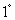Probability Integral (or error function)

``
`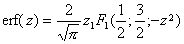`
``I probability integral (or error function)

``Normal probability integral

`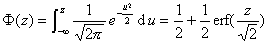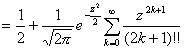`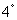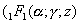Called Kummer function)

[Probability integral asymptotic expression]

`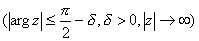`

If the series beforeand term it as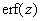an approximation, the error

`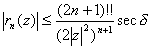`

Whentime is a real number, the error does not exceed the absolute value of the first item in the series omitted.

When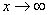when.